Open in App
Not now

## Related Articles

• NCERT Solutions for Class 11 Maths

# Class 11 NCERT Solutions- Chapter 11 Conic Section – Miscellaneous Exercise on Chapter 11

• Last Updated : 12 Mar, 2021

### Question 1. If a parabolic reflector is 20 cm in diameter and 5 cm deep, find the focus.

Solution:

The origin of the coordinate plane is taken at the vertex of the parabolic reflector in such a way that the axis of the reflector is along the positive axis,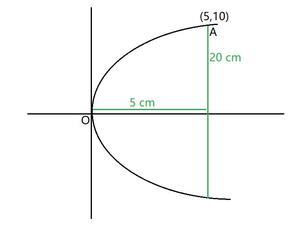The equation for this parabola will be,

y2 = 4ax, which passes through A(5,10)

So, 102 = 4 a(5)

a =a = 5

Hence, the focus of the parabola is (a, 0) = (5, 0), which is the mid – point of the diameter.

### Question 2. An arch is in the form of a parabola with its axis vertical. The arch is 10 m high and 5 m wide at the base. How wide is it 2 m from the vertex of the parabola?

Solution:

We can take the origin of the coordinate plane as vertex of the arch, where its vertical axis is along the positive y – axis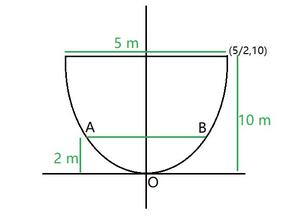The equation for this parabola will be,

x2 = 4ay, which passes through A(,10)

So,= 4 a(10)

4a =4a =Equation of the parabola becomes,

x2y

We need to find width, when height = 2m.

y = 2, so

x2× (2)

x2x =Width = AB

= 2 ×Width (AB) = √5 m

### Question 3. The cable of a uniformly loaded suspension bridge hangs in the form of a parabola. The roadway which is horizontal and 100 m long is supported by vertical wires attached to the cable, the longest wire being 30 m and the shortest being 6 m. Find the length of a supporting wire attached to the roadway 18 m from the middle.

Solution:

According to the given conditions, we can diagrammatically represent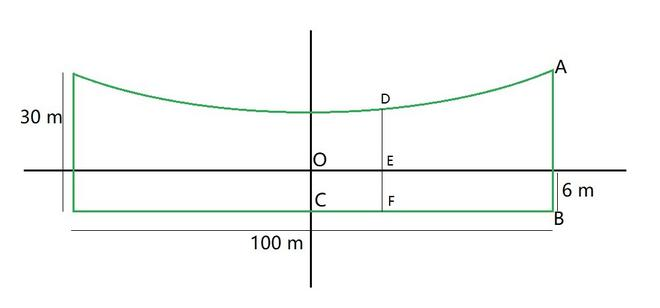Here, AB and OC are the longest and the shortest wires, respectively, attached to the cable.

So, AB = 30m, OC = 6m, and BC = 50m.

The equation for this parabola will be,

x2 = 4ay, which passes through A (50, 30 -6) = (50, 24)

502 = 4a(24)

4a =4a =Equation of the parabola becomes,

x2y

6x2 = 652 y

So, at D where x = 18m

6(18)2 = 652 y

y =y = 3.11 m

DE = 3.11 m

DF = DE + EF = 3.11 +6 = 9.11

Hence, the length of the supporting wire attached to the roadway 18 m from the middle is approximately 9.11 m.

### Question 4. An arch is in the form of a semi-ellipse. It is 8 m wide and 2 m high at the centre. Find the height of the arch at a point 1.5 m from one end.

Solution:

According to the given conditions, we can diagrammatically represent

a = 4m

b = 2m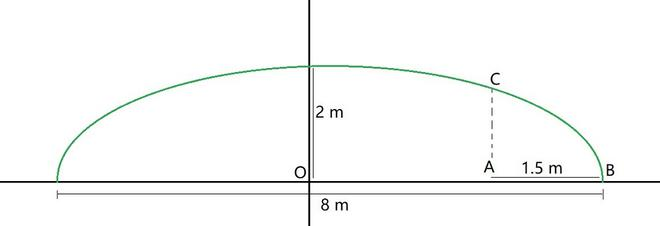The equation of the semi – ellipse will be of the fromLet A be a point on the major axis such that AB = 1.5 m. and AC ⊥ OB.

OA = (4 – 1.5)m = 2.5m

The x – coordinate of point C is 2.5

On substituting x = 2.5, we get,= 1= 1

y2 = 4 (1 –)

= 4 ()

= 2.4375

y = 1.56 (approx.)

So, AC = 1.56 m

Hence, the height of the arch at a point 1.5m from one end is approximately 1.56 m.

### Question 5. A rod of length 12 cm moves with its ends always touching the coordinate axes. Determine the equation of the locus of a point P on the rod, which is 3 cm from the end in contact with the x-axis.

Solution:

According to the given conditions, we can diagrammatically represent,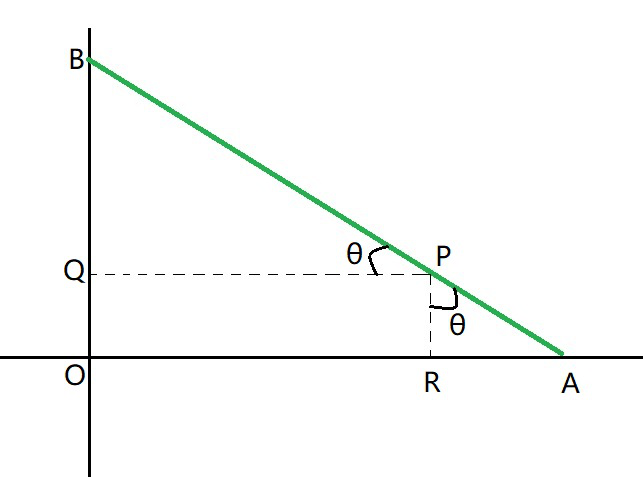Where, let AB be the rod making an angle θ with OX and P(x,y) be the point on it such that

AP = 3cm and AB = 12cm

Then, PB = AB – AP = (12 – 3) = 9cm

From P, draw PQ ⊥ OY and PR ⊥ OX.

In ΔPBQ,

cos θ =Sin θ =we know that, sin2θ +cos2θ = 1,

So,Hence, the equation of the locus of point P on the rod is### Question 6. Find the area of the triangle formed by the lines joining the vertex of the parabola x2 = 12y to the ends of its latus rectum.

Solution:

According to the given conditions, we can diagrammatically represent,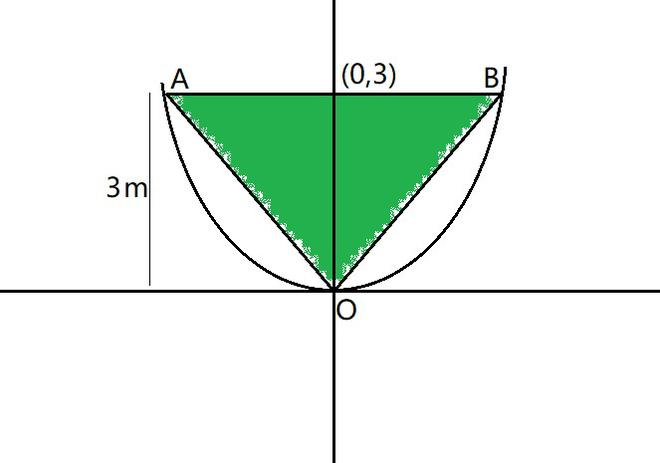The equation for this parabola will be,

x2 = 4ay = 12y

4a = 12

a = 3 cm

Focus is (0,a) = (0,3)

Now let AB be the latus rectum of the given parabola.

At y = 3,

x2 = 12(3)

x2 = 36

x = ±6

So, the coordinates of A are (-6, 3), while the coordinates of B are (6, 3)

Then, the vertices of ΔOAB are O(0,0), A (-6,3) and B(6,3).

By using the formula,

Area of ΔOAB = ½ [0(3-3) + (-6)(3-0) + 6(0-3)]

= ½ [(-6) (3) + 6 (-3)]

= ½ [-18-18]

= ½ [-36]

= 18 sq. unit

Hence, area of ΔOAB is 18 sq. unit

### Question 7. A man running a racecourse notes that the sum of the distances from the two flag posts from him is always 10 m and the distance between the flag posts is 8 m. Find the equation of the posts traced by the man.

Solution:

Let A and B be the positions of the two flag posts and P(x, y) be the position of the man.

So, PA + PB = 10.

We know that if a point moves in plane in such a way that the sum of its distance from two fixed point is constant, then the path is an ellipse and this constant value is equal to the length of the major axis of the ellipse.

Then, the path described by the man is an ellipse where the length of the major axis is 10m, while points A and B are the foci.

2a = 10

Now let us take the origin of the coordinate plane as the centre of the ellipse, and taking the major axis along the x- axis,

According to the given conditions, we can diagrammatically represent,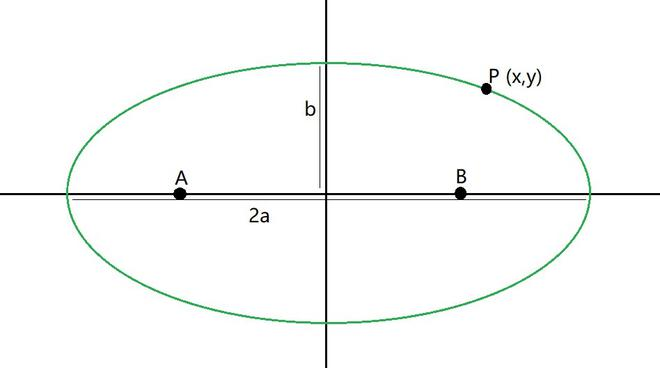The equation for this ellipse will be,, where ‘a’ is the semi-major axis.

So, 2a = 10

a = 5

Distance between the foci,

2c = 8

c = 4

By using the relation, we get

c = √(a2 – b2

4 = √(25 – b2)

16 = 25 – b2

b2 = 25 -16 = 9

Hence, equation of the path traced by the man is### Question 8. An equilateral triangle is inscribed in the parabola y2 = 4 ax, where one vertex is at the vertex of the parabola. Find the length of the side of the triangle.

Solution:

According to the given conditions, we can diagrammatically represent,

Let AB intersect the x – axis at point C.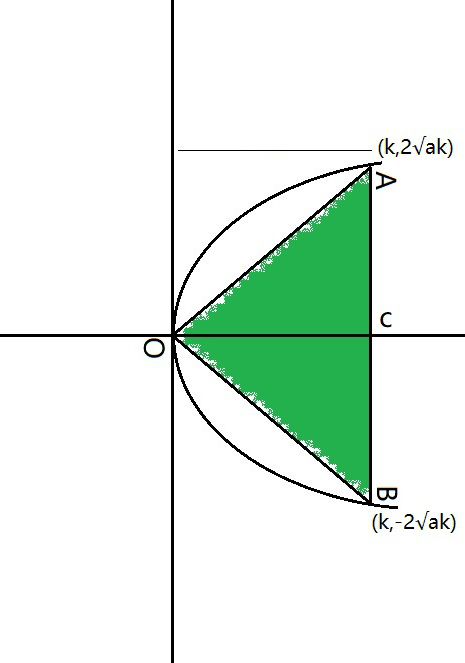Now let OC = k

The equation for this parabola for x = k,

y2 = 4ak

y = ±2√ak

The coordinates of points A and B are (k, 2√ak), and (k, -2√ak)

AB = CA + CB

= 2√ak + 2√ak

= 4√ak

So, OA2 = OC2 + AC2 (Pythagoras theorem)

OA2 = k2 + (2√ak)2

Since, OAB is an equilateral triangle, OA2 = AB2.

k2 + (2√ak)2 = (4√ak)2

k2 + 4ak = 16ak

k2 = 12ak

k = 12a

So, AB = 4√ak = 4√(a×12a)

= 4√12a2

= 4√(4a2×3)

= 8a√3

Hence, the side of the equilateral triangle inscribed in parabola y2 = 4ax is 8a√3 units.

My Personal Notes arrow_drop_up